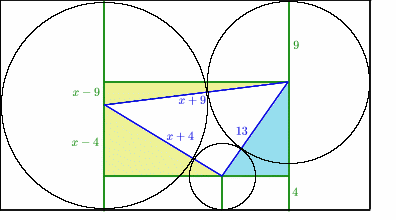#### You may also like### Some(?) of the Parts

A circle touches the lines OA, OB and AB where OA and OB are perpendicular. Show that the diameter of the circle is equal to the perimeter of the triangle### Triangle Incircle Iteration

Keep constructing triangles in the incircle of the previous triangle. What happens?### Circumspection

M is any point on the line AB. Squares of side length AM and MB are constructed and their circumcircles intersect at P (and M). Prove that the lines AD and BE produced pass through P.

# Medallions

##### Age 14 to 16 Challenge Level:

This solution was sent in by Liwei Deng, aged 17, of The Latymer School in London.

First of all, join up the centres of the three circles. This will form a triangle with side lengths $x+9$, $x+4$ and $13$.
Then draw vertical and horizontal lines as shown to create three right-angled triangles (shaded on diagram).
Taking the unknown radius to be $x$, we can mark on the lengths shown in green.We can now use Pythagoras' Theorem on the two yellow right-angled triangles to find the missing sides. The upper one is $6 \sqrt{x}$,

and the lower one is $4 \sqrt{x}$ .

Now we move to the pale blue shaded triangle.
The horizontal side can be found by subtracting:

$$6\sqrt{x} - 4\sqrt{x} = 2\sqrt{x}.$$

The vertical side can also be found by subtracting: as we know that the height of the rectangle is $2x$, this side must be $2x-9-4 = 2x-13$.
Using Pythagoras' Theorem on this triangle, we find that

$$(2\sqrt{x})^2 + (2x-13)^2 = 13^2$$ $$4x + 4x^2 - 52x + 169 = 169$$ $$4x^2 - 48x = 0$$ $$4x(x - 12) = 0$$ $$x = 12$$.